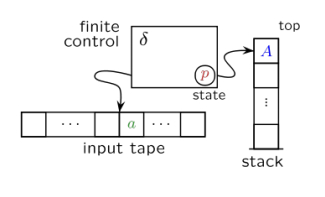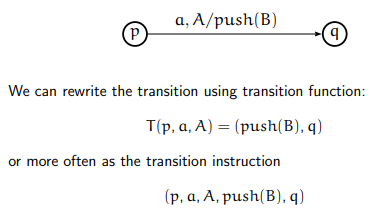# Explain non-deterministic push down automata in TOC?

The Non-deterministic Push down Automata (NPDAs) are like finite automata (FA), except they also have a stack memory where they can store an arbitrary amount of information.Read/write stack memory works as LIFO: Last In, First Out

What can we do with a stack?

The pop operation reads the top symbol and removes it from the stack, the push operation writes a designated symbol onto the top of the stack, e.g. push(X) means put X on top of the stack, the nop operation does nothing to the stack.

The stack symbols are different from the “language” alphabet used on the input tape.

• With only the initial stack symbol on the stack, (nothing else on the stack!) in the start state of the control automaton.

• At each step, the state, input element and top symbol in the stack determine the next step (transition).

One transition step includes the following −

• Change the state, (as FA).

• Reading of a symbol from the input tape and moving to the next right symbol,(as FA).

• Change the stack (pushing a symbol onto the stack/popping a symbol of the stack/no changes to the stack).

Transition steps are formally defined by transition functions (often in the form of the transition instructions).

NPDA can be described as the following −

• A finite set Q of states (& the start state & the set of accepting/final states).

• A finite set ∑ which is called the input alphabet.

• A finite set Γ which is called the stack alphabet (& the initial stack symbol \$).

• A finite set of transition instructions (or a transition function T).

T : Q × ∑ ∪ {∧} × Γ → Γ* × Q

Or it is represented by ‘transition’ diagram as given below −